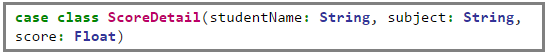# Apache Spark combineByKey Explained

Last updated on May 22,2019 30.4K Views﻿Prithviraj has spent close to two decades in the software development industry...### myMock Interview Service for Real Tech Jobs### myMock Interview Service for Real Tech Jobs

• Mock interview in latest tech domains i.e JAVA, AI, DEVOPS,etc
• Get interviewed by leading tech experts
• Real time assessment report and video recording

Contributed by Prithviraj Bose

Spark is a lightning-fast cluster computing framework designed for rapid computation and the demand for professionals with Apache Spark and Scala Certification is substantial in the market today. Here’s a powerful API in Spark which is combineByKey.

Python API: pyspark.RDD.combineByKey.

The API takes three functions (as lambda expressions in Python or anonymous functions in Scala), namely,

1. Create combiner function: x
2. Merge value function: y
3. Merge combiners function: z

and the API format is combineByKey(x, y, z).

Let’s see an example (in Scala).The full Scala source can be found.

Our objective is to find the average score per student.

Here’s a placeholder class ScoreDetail storing students name along with the score of a subject.Some test data is generated and converted to key-pair values where key = Students name and value = ScoreDetail instance.Then we create a Pair RDD as shown in the code fragment below. Just for experimentation, I have created a hash partitioner of size 3, so the three partitions will contain 2, 2 and 4 key value pairs respectively. This is highlighted in the section where we explore each partition.Now we can explore each partition. The first line prints the length of each partition (number of key value pairs per partition) and the second line prints the contents of each partition.And here’s the finale movement where we compute the average score per student after combining the scores across the partitions.The above code flow is as follows…
First we need to create a combiner function which is essentially a tuple = (value, 1) for every key encountered in each partition. After this phase the output for every (key, value) in a partition is (key, (value, 1)).

Then on the next iteration the combiner functions per partition is merged using the merge value function for every key. After this phase the output of every (key, (value, 1)) is (key, (total, count)) in every partition.

Finally the merge combiner function merges all the values across the partitions in the executors and sends the data back to the driver. After this phase the output of every (key, (total, count)) per partition is
(key, (totalAcrossAllPartitions, countAcrossAllPartitions)).

The map converts the
(key, tuple) = (key, (totalAcrossAllPartitions,  countAcrossAllPartitions))
to compute the average per key as (key, tuple._1/tuple._2).

The last line prints the average scores for all the students at the driver’s end.

Got a question for us? Mention them in the comment section and we will get back to you.

Related Posts:

Get Started with Apache Spark and Scala

Demystifying Partitioning in SparkREGISTER FOR FREE WEBINARThank you for registering Join Edureka Meetup community for 100+ Free Webinars each month